Solving Trigonometric Equations

Remember: The calculator defaults to Radian measure.

 Problem: Find all values of x for which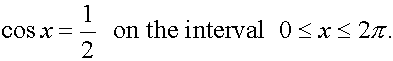Since this problem states the interval in terms of radians, the answer will also need to be expressed in radians.

Let's look at two possible solution methods.  The first method will work ONLY with Radians.  The second method will work with Degrees and then convert back to Radians for the final answer.  Pick the method with which you feel most comfortable.

You are going to graph each side of the equation and look for all of the points of intersection within the given interval.
 Set the MODE to RadianSet Y1 = left side of equation. Set Y2 = right side of equation.Start by hitting ZOOM #7 ZTrig. Adjust WINDOW for Xmin = 0 and Xmax = 2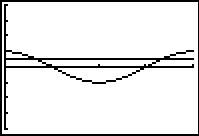Use the INTERSECT option. 2nd TRACE (CALC) #5 intersect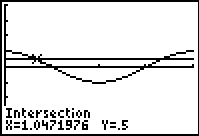To express this answer in terms of, go to Home Screen and hit x ENTER. Divide by.  Convert to fraction. MATH #1 ►Frac.  ENTER.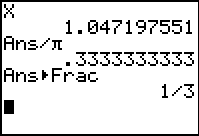Answer: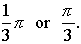Now, find the second point of intersection!Use the INTERSECT option again. 2nd TRACE (CALC) #5 intersect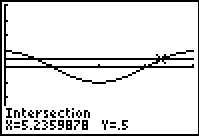To express this answer in terms of, go to Home Screen and hit x ENTER. Divide by.  Convert to fraction. MATH #1 ►Frac.  ENTER.Answer: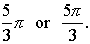Method Using Degree and Converting Back to Radian:

Again, you are going to graph each side of the equation and look for all of the points of intersection within the given interval.
For this method, you must be aware that the interval in degrees is 0 < x < 360.

 Set the MODE to Degree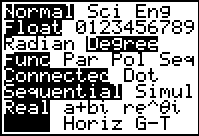Set Y1 = left side of equation. Set Y2 = right side of equation.Start by hitting ZOOM #7 ZTrig. Adjust WINDOW for Xmin = 0 and Xmax = 360Use the INTERSECT option. 2nd TRACE (CALC) #5 intersectTo convert to radians (in terms of), go to Home Screen and hit x/180  ENTER.  Convert to fraction. MATH #1 ►Frac.  ENTER.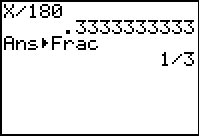Answer:Now, find the second point of intersection!Use the INTERSECT option again. 2nd TRACE (CALC) #5 intersect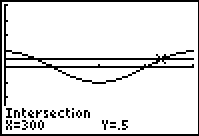To convert to radians (in terms of), go to Home Screen and hit x /180 ENTER.  Convert to fraction. MATH #1 ►Frac.  ENTER.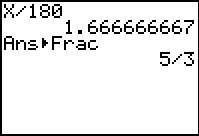Answer: